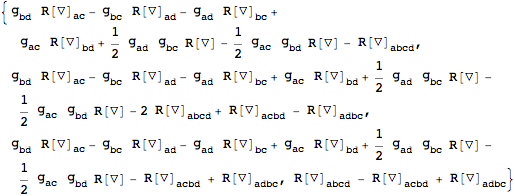xTras Package Symbol

# ConstructDDIs

 ConstructDDIs[expr]constructs all scalar dimensional dependent identities that can be build out of expr. ConstructDDIs[expr, frees]constructs all dimensional dependent identities that can be build out of expr and that have free indices frees. ConstructDDIs[expr, frees, sym]constructs all dimensional dependent identities that can be build out of expr and that have the symmetry sym imposed on their free indices frees.
• ConstructDDIs returns a list of tensorial expressions that are zero due to over-antisymmetrization.
• ConstructDDIs uses AllContractions internally, hence its conditions on the input expression also apply to ConstructDDIs.
• The number of indices of expr plus the number of additional frees has to be at least, whereis the dimension of the manifold.
• ConstructDDIs computes all possible contractions between expr and the basic dimensional dependent identity, BasicDDI.
• ConstructDDIs defines the BasicDDI the first time it's called.
• ConstructDDIs only works when the dimension of the manifold is a non-generic integer.
• It is possible to change the dimension of the manifold in between ConstructDDIs calls.
Vanishing of the Einstein tensor in 2 dimensions:
Vanishing of the Weyl tensor in 3 dimensions:
Vanishing of the Einstein tensor in 2 dimensions:
 In:=
 Out=In:=Out=Vanishing of the Weyl tensor in 3 dimensions:
 In:=
 Out=In:=Out=In:=
 Out=When the dimension of the manifold is not an integer, ConstructDDIs doesn't work:
The number of indices of the input expression has to be at least, where d is the dimension of the manifold.